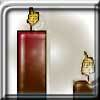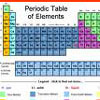#### You may also like### Burning Down

One night two candles were lit. Can you work out how long each candle was originally?### Fair Shares?

A mother wants to share a sum of money by giving each of her children in turn a lump sum plus a fraction of the remainder. How can she do this in order to share the money out equally?### A Method of Defining Coefficients in the Equations of Chemical Reactions

A simple method of defining the coefficients in the equations of chemical reactions with the help of a system of linear algebraic equations.

# A Problem of Time

##### Age 14 to 16Challenge Level

Tim sent us his excellent answer to this problem:

The time is approximately 27 minutes 42 seconds past 6 o'clock.

At six o'clock the minute hand and the hour hand are exactly 180 degrees apart. Let the hour hand move through x degrees. Then, if the time is reversible in a mirror, the minute hand has moved through (180 - x) degrees. The hour hand moves at 30 degrees per hour. The minute hand moves at 360 degrees per hour. The time elapsed during which both hands move is identical. It follows that
$\frac{(180 - x)}{360} = \frac{x}{30}$
x = $\frac{180}{13}$

Hence, this angle represents $\frac{180}{13}$ x $\frac{1}{360}$ x 60 minutes on the clock face. This reduces to 2$\frac{4}{13}$ minutes (approx. 2 minutes and 18 seconds). Therefore the time is 30 - 2$\frac{4}{13}$ minutes past 6 o'clock; i.e. 27$\frac{9}{13}$ minutes past 6 o'clock.

Laura (West Flegg Middle School, Great Yarmouth, Norfolk) came very close to this answer. Her solution was 28 minutes past 6 o'clock.